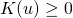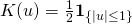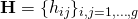# Kernel based Estimators for Multivariate Densities and Functions

## 1. Kernel Functions

In general, a kernelis an integrable function  satisfying

1.2.is symmetric (e.g.if)
3..

Popular univariate () kernel functions:

• Uniform:• Epanechnikov:• Gaussian:An easy way to construct a multivariate (g>1) kernel from an univariate kernel is to construct a product kernel. Letandbe an univariate kernel thenAn important feature is to scale kernel functions by a parameter matrixwith.

## 2. Kernel Density Estimation

A famous application for kernels is to estimate the underlining density functions of a given independent and identically distributed-variate random vectorsdrawn from some distribution with an unknown density. A kernel based density estimator is then given byA naive Matlab implementation is straightforward:

% ------------------------------------------------------------------------------
% Description: g-Dimensional Density Estimator
%
% ------------------------------------------------------------------------------
% Usage:       -
% ------------------------------------------------------------------------------
% Inputs Simulation:
%X    - observations (Dimension: Txg)
%x    - g dimensional output coordinates (Dimension: T_outxg)
%H    - Bandwidth matrix (Dimension: gxg)
%type - Kernel choice, 'Gauss' for gaussian kernel, Epanechnikov else.

% ------------------------------------------------------------------------------
%Output:
%fit  - Estimated Density

% ------------------------------------------------------------------------------
% Keywords:    kernel, density estimation
% ------------------------------------------------------------------------------
% ------------------------------------------------------------------------------
% Author:      Heiko Wagner, 2017/01/18
% ------------------------------------------------------------------------------

function [fit] = density(X,x,H,k_type)
T = size(X,1);
T_out = size(x,1);

%% Epanechnikov Kernel function
function [k]=epan(t);
k= 3/4*(1-t.^2);
k((abs(t)-1)&gt;0)=0;
end

%%Construct Productkernel
function [A]=kernel(Xn)
g=size(Xn,2);
A=1;
if(strcmp(k_type,'Gauss')==1)   %Use Gaussian Kernel
for (m=1:g)
A= A.*normpdf(Xn(:,m))  ;
end
else                            %Use Epanechnikov Kernel
for (m=1:g)
A= A.*epan(Xn(:,m))  ;
end
end
end

Xn=zeros( size(X) );
function [f_j]=getpoint(x_j)
%%%Construct g dimensional grid around point j, at this pint you can improve the code if you use the Epanechnikov Kernel by only selecting certain observations
Xn = X - repmat( x_j,T,1 );
f_j= 1/T*det(H)^(-1)* sum( kernel(Xn*H^(-1)) )
end
%%%To estimate not just a single point we estimate the function for an entire set of g dimensional output coordinates (x)

[out]=arrayfun(@(s) getpoint( x(s,:) ), 1:T_out , 'UniformOutput', false);
fit=[out{:}]';
end 

The run time of this an algorithm in-notation, that evaluates the density atpoints, is then given by. Can we do better? Yes, we can. A smarter way is to make use of the fast-fourier transformation (FFT) introduced by .

 B. W. Silverman, “Algorithm as 176: kernel density estimation using the fast fourier transform,” Journal of the royal statistical society. series c (applied statistics), vol. 31, iss. 1, p. 93–99, 1982.
[Bibtex]
@article{Silverman1982,
ISSN = {00359254, 14679876},
URL = {http://www.jstor.org/stable/2347084},
author = {B. W. Silverman},
journal = {Journal of the Royal Statistical Society. Series C (Applied Statistics)},
number = {1},
pages = {93--99},
publisher = {[Wiley, Royal Statistical Society]},
title = {Algorithm AS 176: Kernel Density Estimation Using the Fast Fourier Transform},
volume = {31},
year = {1982}
}

### 3 thoughts on “Kernel based Estimators for Multivariate Densities and Functions”

This site uses Akismet to reduce spam. Learn how your comment data is processed.